June 27, 2016

## Goal

• Out-of-the-box methodology applicable to experience sampling method (ESM) data
• Multiple people measured multiple times in relatively short time span (several weeks)
• Up to three network structures can be obtained in ESM data:
• Contemporaneous networks
• Temporal networks
• Between-subjects networks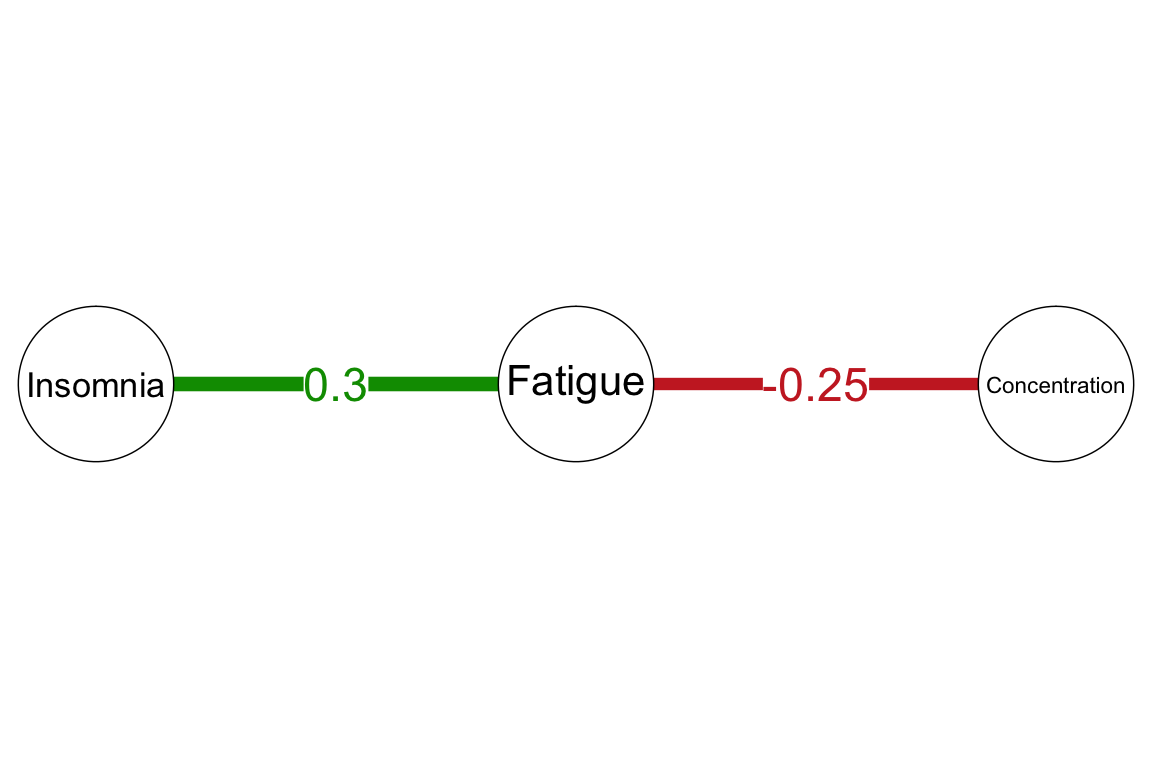• The Gausian graphical models the inverse variance-covariance matrix
• $$\pmb{K} = \pmb{\Sigma}^{-1}$$
• Network of partial correlation coefficients:
• $$\mathrm{Cor}\left(Y_i,Y_j \mid \pmb{Y}^{-(i,j)}\right) = - \frac{\kappa_{ij}}{\sqrt{\kappa_{ii}} \sqrt{\kappa_{jj}}}$$

The GGM model:

• Concentration $$-$$ Fatigue $$-$$ Insomnia

Is equivalent to three causal structures:

1. Concentration $$\rightarrow$$ Fatigue $$\rightarrow$$ Insomnia
2. Concentration $$\leftarrow$$ Fatigue $$\rightarrow$$ Insomnia
3. Concentration $$\leftarrow$$ Fatigue $$\leftarrow$$ Insomnia

Thus, the GGM highlights potential causal pathways. In addition, the partial correlations are proportional to multiple regression coefficients.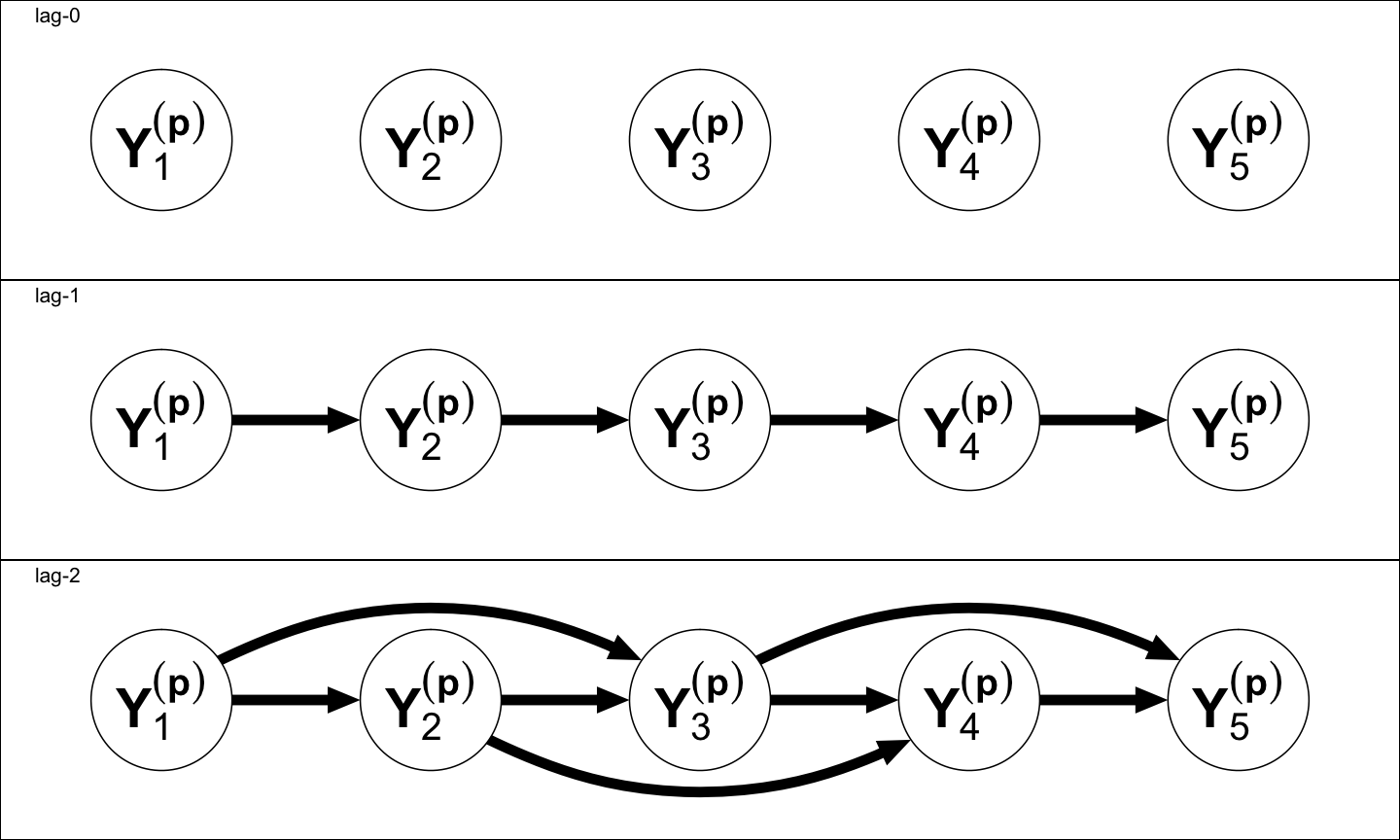• We will use the lag-1 factorization

## Vector Auto-regression

$\pmb{Y}_t \mid \pmb{y}_{t-1} \sim N\left( \pmb{\mu} + \pmb{B} \left(\pmb{y}_{t-1} - \pmb{\mu}\right), \pmb{\Theta} \right)$

• $$\pmb{B}$$ encodes the temporal network
• Granger causality
• $$\pmb{\Theta}^{-1}$$ encodes the contemporaneous network
• GGM
• The sample means can be used as plugin to center the predictors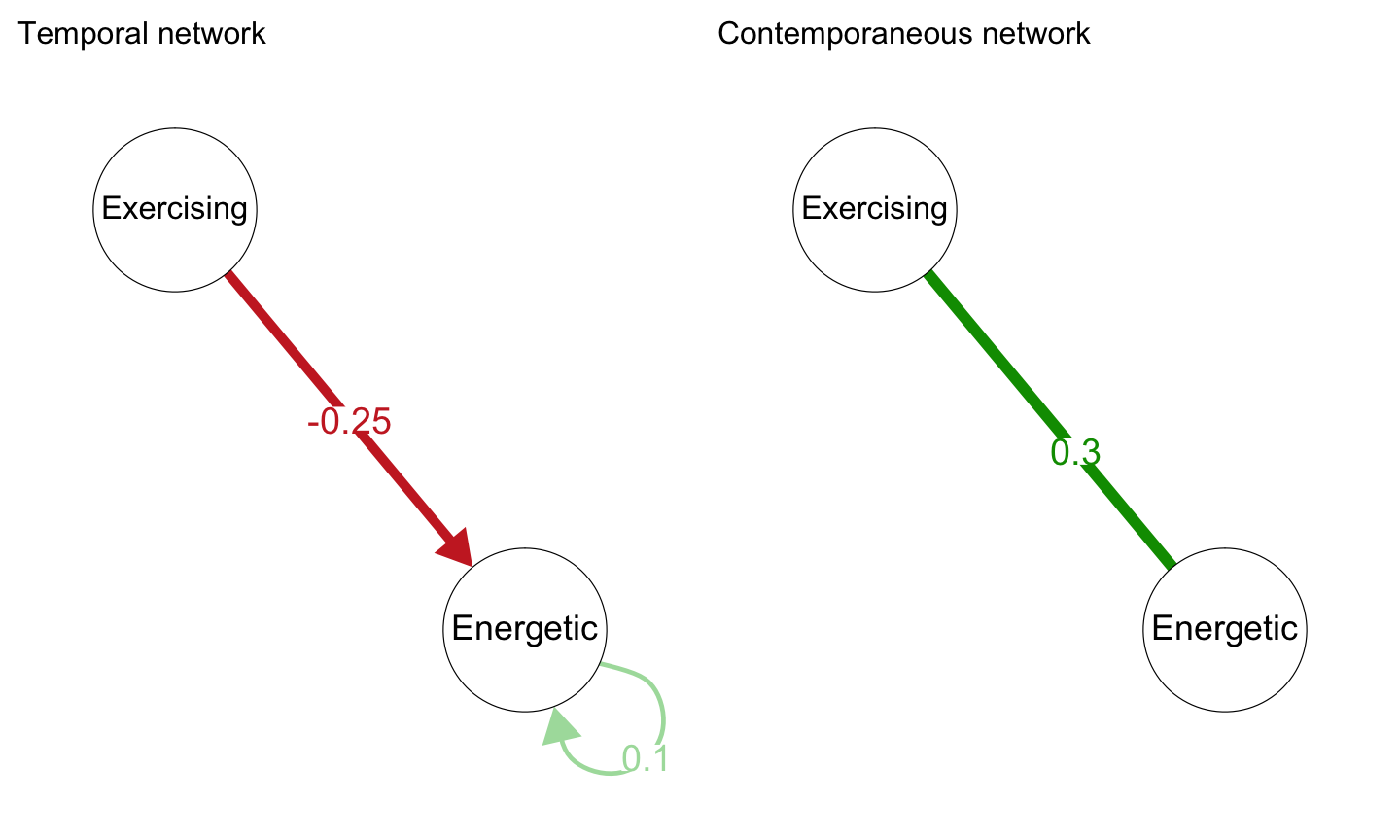## Contemporaneous Causation

• Many causal effects likely faster than the time-window of measurement
• Somatic arousal $$\rightarrow$$ anticipation of panic attack $$\rightarrow$$ anxiety
• These can be caught in a contemporaneous network of partial correlations
• Thus, the contemporaneous network can also be seen to highlight potential causal relationships
• As the contemporaneous network is the GGM, the temporal network can be seen as a correction for dependent measurements in estimating the GGM

## Empirical Example

Data collected by Date C. Van der Veen, in collaboration with Harriette Riese en Renske Kroeze.

• Patient suffering from panic disorder and depressive symptoms
• Perfectionist
• Measured over a period of two weeks
• Five times per day
• Items were chosen after intake together with therapist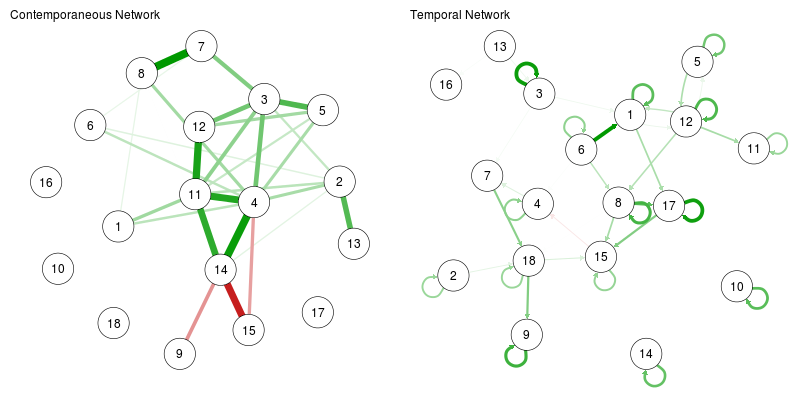1: anxious, 2: stressed, 3: angry, 4: sad, 5: guilty, 6: weak, 7: worthless, 8: helpless, 9: full of energy, 10: fear panic attack, 11: fear to cry, 12: fear to appear angry, 13: 'had to do things', 14: bodily discomfort, 15: enjoying, 16: let something pass, 17: social env pleasurable, 18: physically active

## Multi-level VAR

Adding superscript $$p$$ for subject. Level 1 model: $\pmb{Y}^{(p)}_t \mid \pmb{y}^{(p)}_t = N\left(\pmb{\mu}^{(p)} + \pmb{B}^{(p)} \left(\pmb{y}_{t-1}^{(p)} - \pmb{\mu}^{(p)} \right), \pmb{\Theta}^{(p)} \right)$

Level 2 model: $\begin{bmatrix} \pmb{R}_{\pmb{\mu}} \\ \pmb{R}_{\pmb{B}} \end{bmatrix} \sim N\left(\pmb{0}, \begin{bmatrix} \pmb{\Omega}_{\pmb{\mu}} & \pmb{\Omega}_{\pmb{\mu}\pmb{B}} \\ \pmb{\Omega}_{\pmb{B}\pmb{\mu}} & \pmb{\Omega}_{\pmb{B}} \end{bmatrix} \right).$

• Block $$\pmb{\Omega}_{\pmb{\mu}}$$ encodes the between-subject relationships between means
• These can be used to estimate a GGM
• Between-subjects network of partial correlations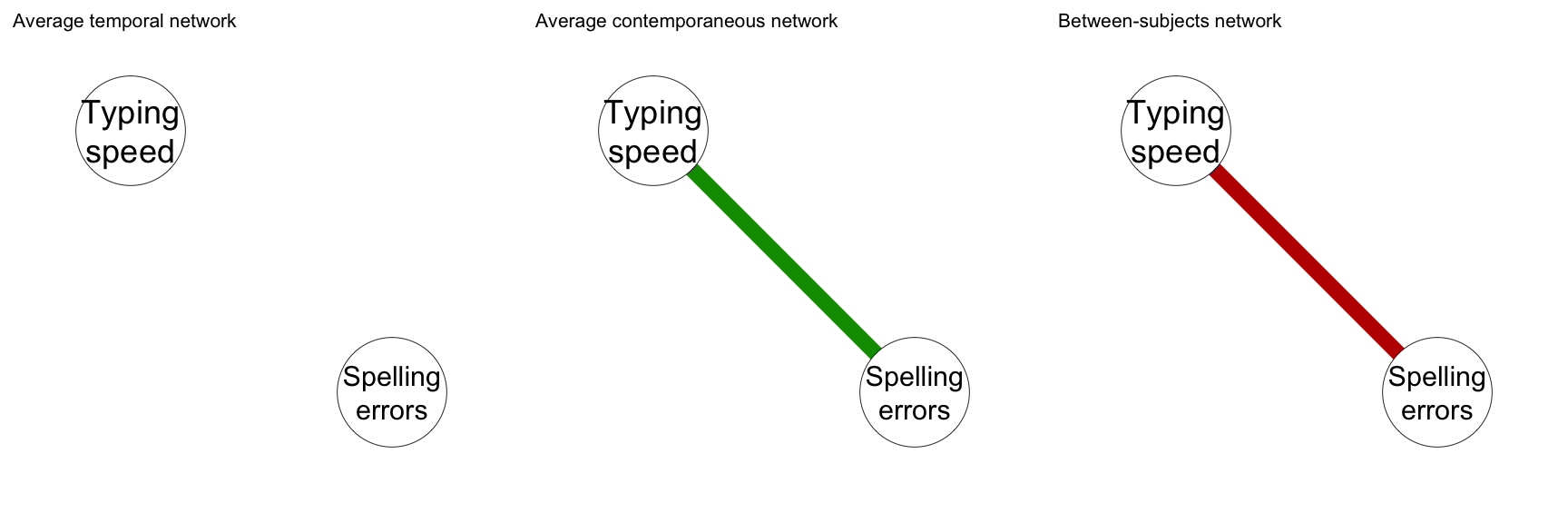Example based on Hamaker, E. L. (2012). Why Researchers Should Think 'Within-Person': A Paradigmatic Rationale. Handbook of Research Methods for Studying Daily Life. The Guilford Press New York, NY, 43–61.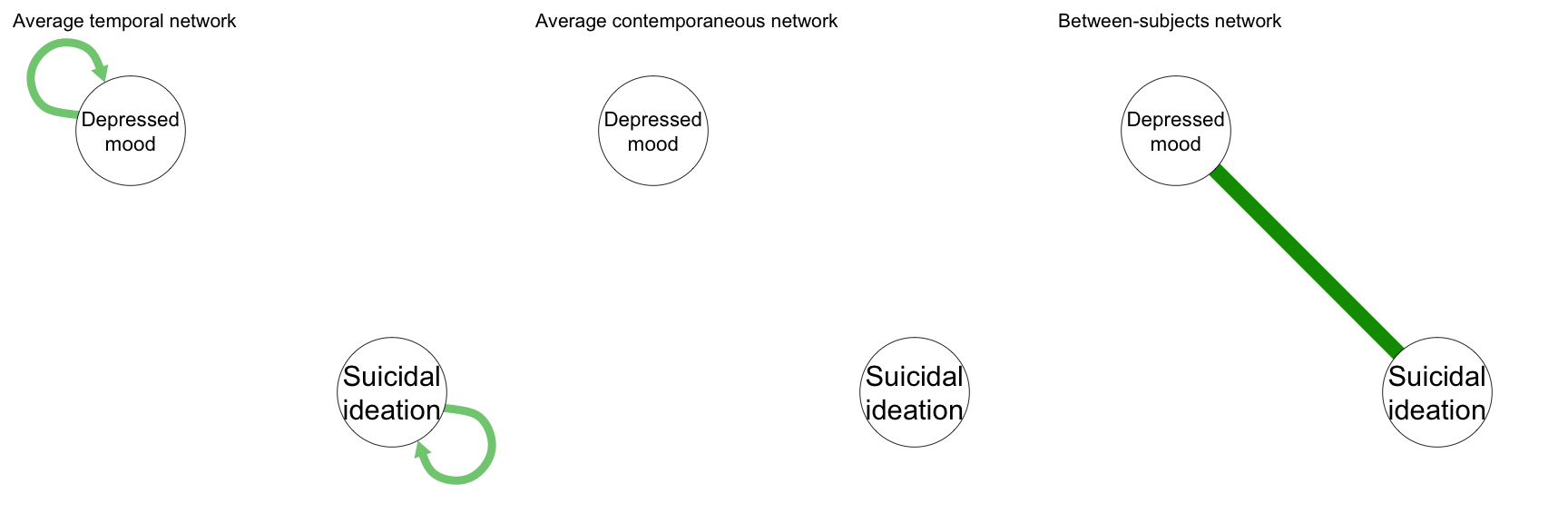Hypothetical example of networks based on two persons:

• Clinically depressed person constantly scoring high on both
• Healthy person constantly scoring low on both

## Empirical Example

• Two datasets
• Original: 26 subjects, 51 measurements on average, 1323 total observations
• Replication: 65 subjects, 35.5 measurements on average, 2309 total observations
• 16 indicators of neuroticism, extroversion, conscientiousness
• Orthogonal estimation of temporal and contemporaneous effects
• Only significant effects shown
• Alpha = 0.05 and using the "or" rule
• Very preliminary results
• I ran the analysis two days ago!